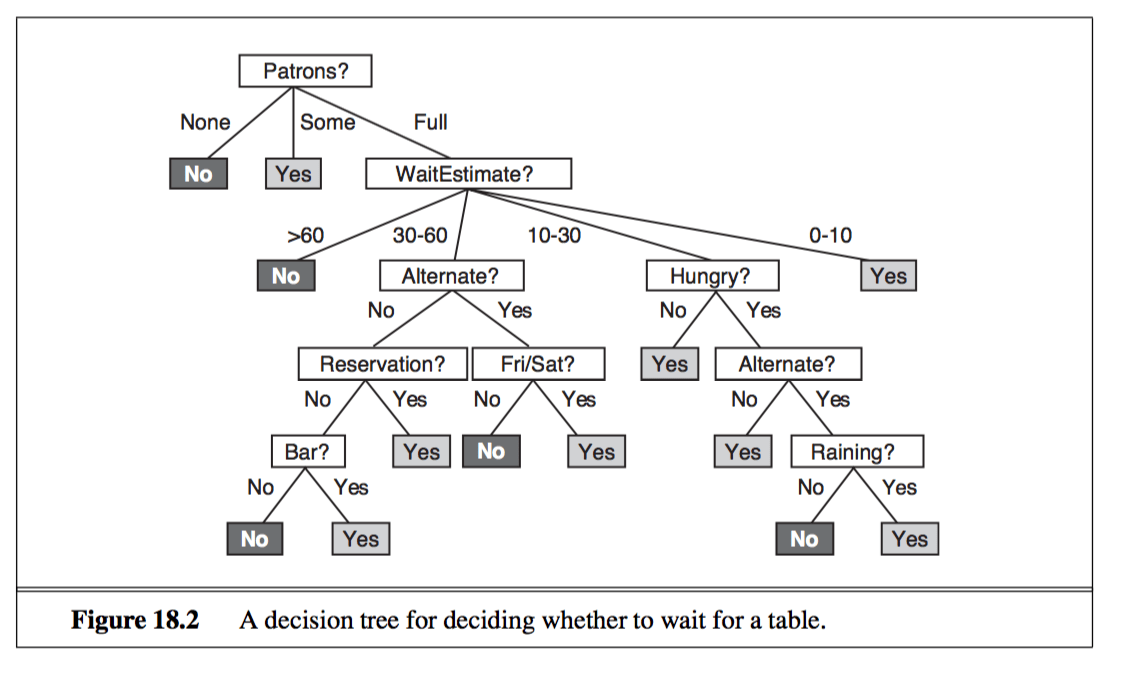# Drawing graphs

Notice how nice some of the graphs are in textbooks (for example, from AIMA 3rd ed.)?Well you could draw them too with graphviz (dot language).``` digraph G { rankdir=LR splines=line node [fixedsize=true, label=""]; subgraph cluster_0 { color=white; node [style=solid,color=blue4, shape=circle]; x1 x2 x3; label = "layer 1 (Input layer)"; } subgraph cluster_1 { color=white; node [style=solid,color=red2, shape=circle]; a12 a22 a32; label = "layer 2 (hidden layer)"; } subgraph cluster_2 { color=white; node [style=solid,color=seagreen2, shape=circle]; O; label="layer 3 (output layer)"; } x1 -> a12; x1 -> a22; x1 -> a32; x2 -> a12; x2 -> a22; x2 -> a32; x3 -> a12; x3 -> a22; x3 -> a32; a12 -> O a22 -> O a32 -> O } ```